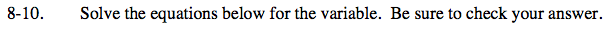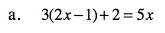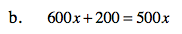Home > ACC7 > Chapter cc28 > Lesson cc28.1.1 > Problem8-10

8-10.
1. Solve the equations below for the variable. Be sure to check your answer. Homework Help ✎

1. 3(2x − 1) + 2 = 5x

2. 600x + 200 = 500xDistrubute.

Combine like terms.

Subtract 5x from each side.

Add 1 to each side.

3(2x) − 3(1) + 2 = 5x

6x − 3 + 2 = 5x

6x − 1 = 5x

−5x + 6x − 1 = 5x −5x
x − 1 = 0

x − 1 +1 = 0 +1 +1

x = 1Divide each term by 100.

Substitute 1 back into the original equation to check your answer.

3(2(1) − 1) + 2 = 5(1)

When you simplify this expression, do you get the same values on each side?